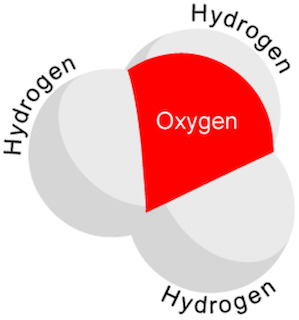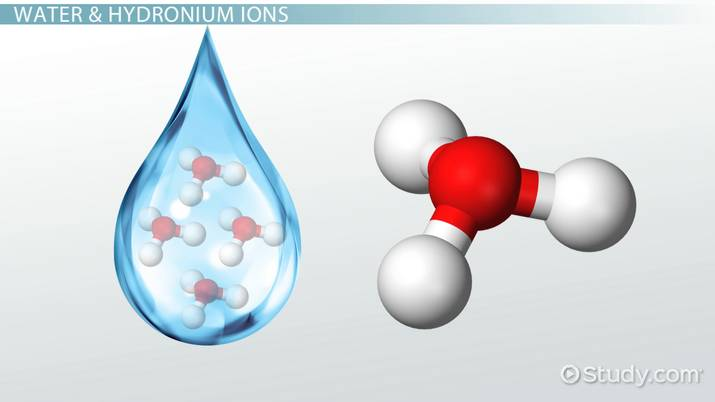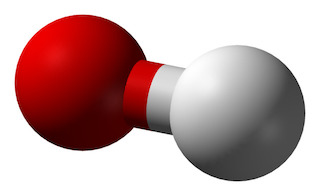# Hydronium Ion

chase smith, Emily Lockhart
• Author
chase smith

Chase is a 14 year veteran science teacher with a specialization is chemistry/STEM. He has a Bachelors Degree in Chemistry and a Master's Degree in Instructional Media with a specialization in STEM.

• Instructor
Emily Lockhart

Emily has taught science and has a master's degree in education.

Learn about the hydronium cation, which has the chemical formula H3O+. Understand the definition of hydronium ion and the formula for calculating hydronium ions. Updated: 12/01/2021

Show

## Hydronium Cation

A hydrodium ion is best thought of as a water molecule, combined with a positively charged hydrogen ion. In the case of a water molecule combining with a hydrogen ion, the resulting equation can be seen below:

{eq}H_{2}O\: + \: H^{+}\: \: \rightarrow \: \: H_{3}O^{+} {/eq}

The product of {eq}H_{3}O^{+} {/eq} is referred to as the hydronium ion. Due to its positive charge, hydronium is classified as a cation. Cations have achieved their positive charge by donating an electron to a solution, leaving the cation having fewer electrons than it has protons. This lack of electrons leads to a positive overall charge. Any time a neutral atom gains or loses electrons, the resulting particle is referred to as an ion.

### Hydronium Ion Definition

A picture of a hydronium ion can be seen here:In this image, it can be seen that there are 3 hydrogen atoms and 1 oxygen. Since oxygen atoms will traditionally form -2 charged ions, and hydrogen typically forms +1 ions, the resulting charge of this combination of atoms equals a +1 charge.

### Water & Hydronium Ions

Water and hydronium ions are very closely related. The only separation between the two of them is a hydrogen ion. A hydrogen ion is nothing more than a proton. It is relatively easy for a hydrogen ion to get attached to a water molecule in a solution creating hydronium. In short, anytime there is a proton that attaches to a water molecule, hydronium is created.An error occurred trying to load this video.

Try refreshing the page, or contact customer support.

Coming up next: Rules for Naming Ionic Compounds

### You're on a roll. Keep up the good work!

Replay
Your next lesson will play in 10 seconds
• 0:04 Water & Hydronium Ions
• 1:15 Origins of an Ion
• 2:05 Hydronium & Hydroxide Ions
• 4:32 Formulas
• 5:37 Lesson Summary
Save Save

Want to watch this again later?

Timeline
Autoplay
Autoplay
Speed Speed

## Hydronium & Hydroxide Ions in Acid Solution

The most common use of the term hydronium is during a discussion on whether solutions are acidic/basic. Hydronium is one of the two key ions that are measured to determine the pH of a solution, with the other being hydroxide. The pH of a solution is nothing more than a calculation done to measure how much hydronium ion is present inside of a solution. The equation below will be a key to help calculate the hydronium ion of a solution:

{eq}H_{3}O^{+}\: x\: OH^{-}\: =\: 1x10^{^{-14}} {/eq}

Both the hydronium and hydroxide ions are referenced in terms of concentrations in this equation. In words, this equation is saying that the hydronium ion concentration multiplied by the hydroxide ion concentration will always equal {eq}1x10^{^{-14}} {/eq}. By having the concentrations of either the hydronium ions or the hydroxide ions, the opposite type of ion's concentrations can be calculated from this equation by rearranging the formula algebraically.## Hydronium Ion Formula: Calculate Hydronium Ions in pH Equation

The pH scale is based on the logarithmic scale. The pH is equal to the negative logarithm of the hydrogen ion concentration. Being on the logarithmic scale means that a pH of 1 is actually 10 times stronger than a pH of 2. They are only a magnitude of 1 apart on the pH scale, but on the log scale, this equates to a factor of 10 difference.

Using math to calculate, the pH of a solution can be calculated using the following equation:

{eq}pH= -log(H^{ +}) {/eq}

The hydrogen ion in this formula is easily replaced with the hydronium ion, as they are interchangeable when referencing acidic solutions.

The log scale makes calculating the pH of a certain concentration of an acid very simple in many circumstances. For example:

To unlock this lesson you must be a Study.com Member.

#### Is H3O+ a cation?

Yes. Hydronium is a cation that has a formula of H3O+. Since it has a positive charge, it classifies as a cation.

#### Is H3O+ the same as H+?

H3O+ is extremely similar to H+, but they should not be considered identically the same thing. H3O+ only is present in aqueous solutions, while H+ ions can occur in several different circumstances.

#### Is H3O+ an acid or base?

H3O+ is the sign of an acid being present. The concentration of H3O+ determines the magnitude of the acidity of a solution.

#### What is the proper name for H3O+?

H3O+ is most commonly referred to as a hydronium ion. It occurs when a hydrogen ion combines with a water molecule.

#### What is the hydronium ion formula?

Hydronium is a cation that has a formula of H3O+. It often is made from a water molecule combined with a positively charged hydrogen ion.

#### Is hydronium a cation?

Yes. Hydronium is a cation that has a formula of H3O+. Since it has a positive charge, it classifies as a cation.

### Register to view this lesson

Are you a student or a teacher?